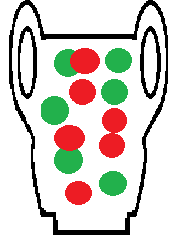# Urn Model: Simple Definition, Examples and ApplicationsUrn models are simple ways to represent real life probabilities.In statistics, an urn model is an idealized way of modeling real-life problems as if they were problems which involve drawing balls out of an urn, or box.

The urn contains balls of two or more colors. Depending on the problem being studied, the balls may be:

• Replaced after each draw (called sampling with replacement),
• Not replaced after each draw (called sampling without replacement),
• Added depending on the outcome of the draw. For example, if you draw a green ball, you might add another green ball to the urn. This is called a Pólya Urn.

## The basic urn model

In the basic urn problem, the urn contains just black and white balls: x black balls and y white balls. A ball is drawn from the urn, its color is recorded, and then the next ball is drawn. The balls may be replaced after their color has been recorded, or they may not be.

• Knowing x and y, what is the probability of a given sequence?
• How long a sequence of white balls do I need to draw to be sure, with a given degree of certainty, that there are no black balls in the urn?
• How many balls do we need to draw to figure out the proportions of black and white balls, to a given degree of certainty?

## Applications of the Urn Model

The binomial distribution, multinomial distribution, geometric distribution and the hypergeometric distribution are just some examples of important probability distributions in statistics that can be modeled on the urn problem.

Since we use these distributions so widely in statistical work, it should come as no surprise that the urn model can be successfully used to model real world processes in fields as diverse as genetics, ecology, physics, and economics.

Related aticles:
The Pólya Urn
Wallenius’ Distribution / Urn

## References

DasGupta A. (2010) Urn Models in Physics and Genetics. In: Fundamentals of Probability: A First Course. Springer Texts in Statistics. Springer, New York, NY.
Balakrishnan, N. (Ed.). Advances in Combinatorial Methods and Applications to Probability and Statistics.

CITE THIS AS:
Stephanie Glen. "Urn Model: Simple Definition, Examples and Applications" From StatisticsHowTo.com: Elementary Statistics for the rest of us! https://www.statisticshowto.com/urn-model/
---------------------------------------------------------------------------Need help with a homework or test question? With Chegg Study, you can get step-by-step solutions to your questions from an expert in the field. Your first 30 minutes with a Chegg tutor is free!